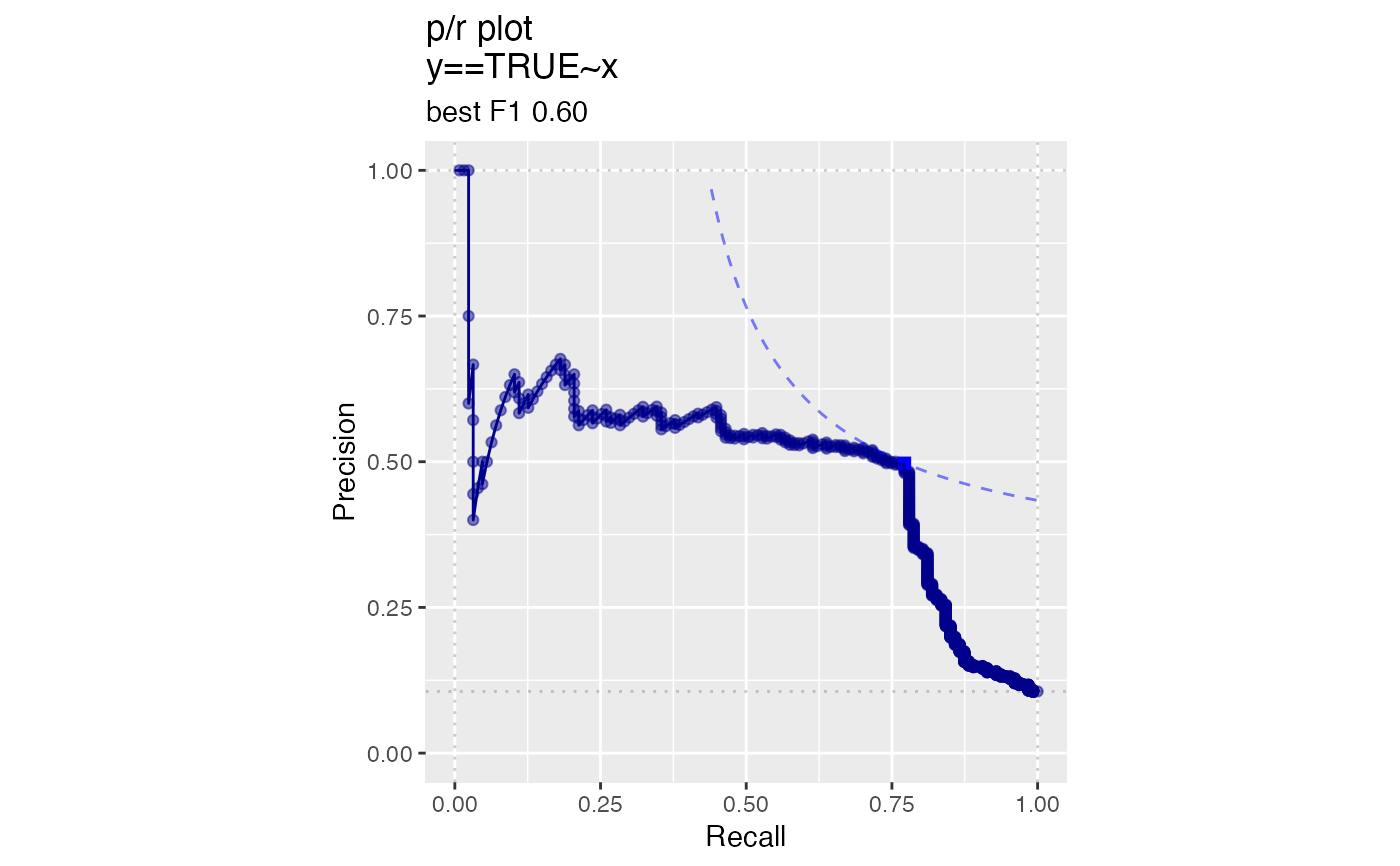Plot classifier metrics as a function of thresholds.

ThresholdPlot(
frame,
xvar,
truthVar,
title,
...,
metrics = c("sensitivity", "specificity"),
truth_target = TRUE,
points_to_plot = NULL,
monochrome = TRUE,
palette = "Dark2",
linecolor = "black"
)

## Arguments

frame data frame to get values from column of scores column of true outcomes title to place on plot no unnamed argument, added to force named binding of later arguments. metrics to be computed. See Details for the list of allowed metrics truth value considered to be positive. how many data points to use for plotting. Defaults to NULL (all data) logical: if TRUE, all subgraphs plotted in same color character: if monochrome==FALSE, name of brewer color palette (can be NULL) character: if monochrome==TRUE, name of line color

## Details

By default, ThresholdPlot plots sensitivity and specificity of a a classifier as a function of the decision threshold. Plotting sensitivity-specificity (or other metrics) as a function of classifier score helps identify a score threshold that achieves an acceptable tradeoff among desirable properties.

ThresholdPlot can plot a number of metrics. Some of the metrics are redundant, in keeping with the customary terminology of various analysis communities.

• sensitivity: fraction of true positives that were predicted to be true (also known as the true positive rate)

• specificity: fraction of true negatives to all negatives (or 1 - false_positive_rate)

• precision: fraction of predicted positives that are true positives

• recall: same as sensitivity or true positive rate

• accuracy: fraction of items correctly decided

• false_positive_rate: fraction of negatives predicted to be true over all negatives

• true_positive_rate: fraction of positives predicted to be true over all positives

• false_negative_rate: fraction of positives predicted to be all false over all positives

• true_negative_rate: fraction negatives predicted to be false over all negatives

For example, plotting sensitivity/false_positive_rate as functions of threshold will "unroll" an ROC Plot.

ThresholdPlot can also plot distribution diagnostics about the scores:

• fraction: the fraction of datums that scored greater than a given threshold

• cdf: CDF or 1 - fraction; the fraction of datums that scored less than a given threshold

Plots are in a single column, in the order specified by metrics.

points_to_plot specifies the approximate number of datums used to create the plots as an absolute count; for example setting points_to_plot = 200 uses approximately 200 points, rather than the entire data set. This can be useful when visualizing very large data sets.

PRTPlot

## Examples


# data with two different regimes of behavior
d <- rbind(
data.frame(
x =  rnorm(1000),
y = sample(c(TRUE, FALSE), prob = c(0.02, 0.98), size = 1000, replace = TRUE)),
data.frame(
x =  rnorm(200) + 5,
y = sample(c(TRUE, FALSE), size = 200, replace = TRUE))
)

# Sensitivity/Specificity examples
ThresholdPlot(d, 'x', 'y',
title = 'Sensitivity/Specificity',
metrics = c('sensitivity', 'specificity'),
truth_target = TRUE)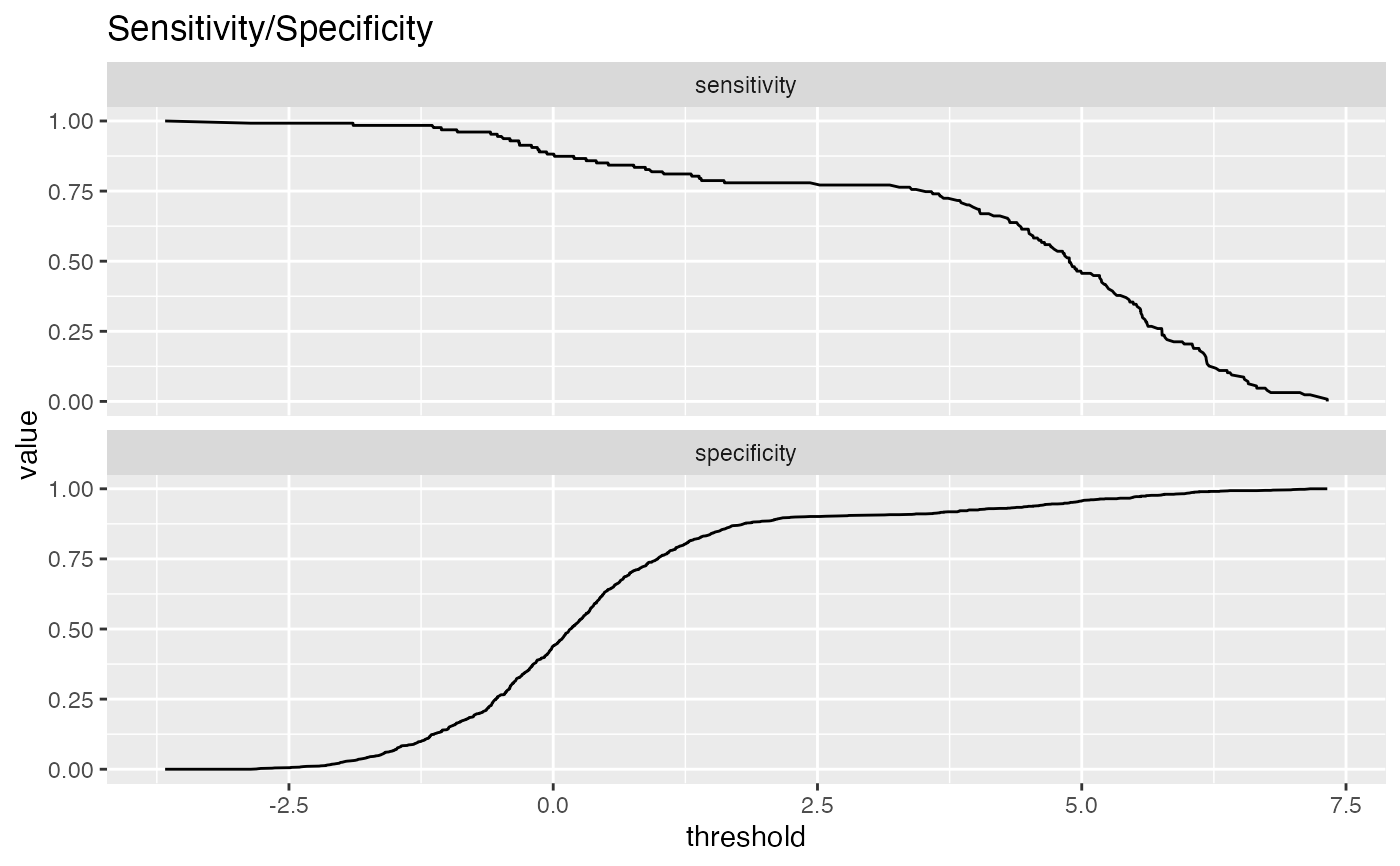MetricPairPlot(d, 'x', 'y',
x_metric = 'false_positive_rate',
y_metric = 'true_positive_rate',
truth_target = TRUE,
title = 'ROC equivalent')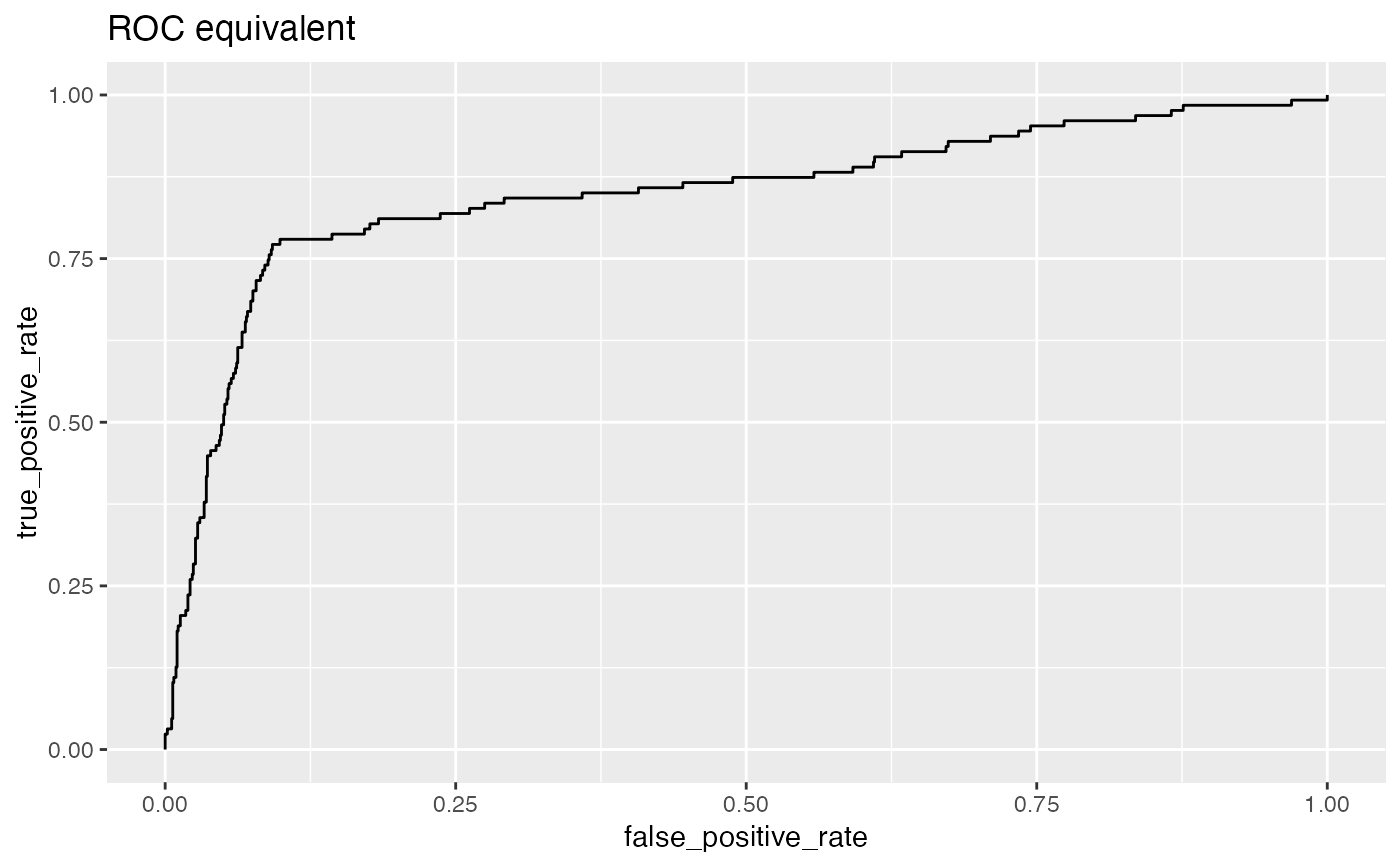ROCPlot(d, 'x', 'y',
truthTarget = TRUE,
title = 'ROC example')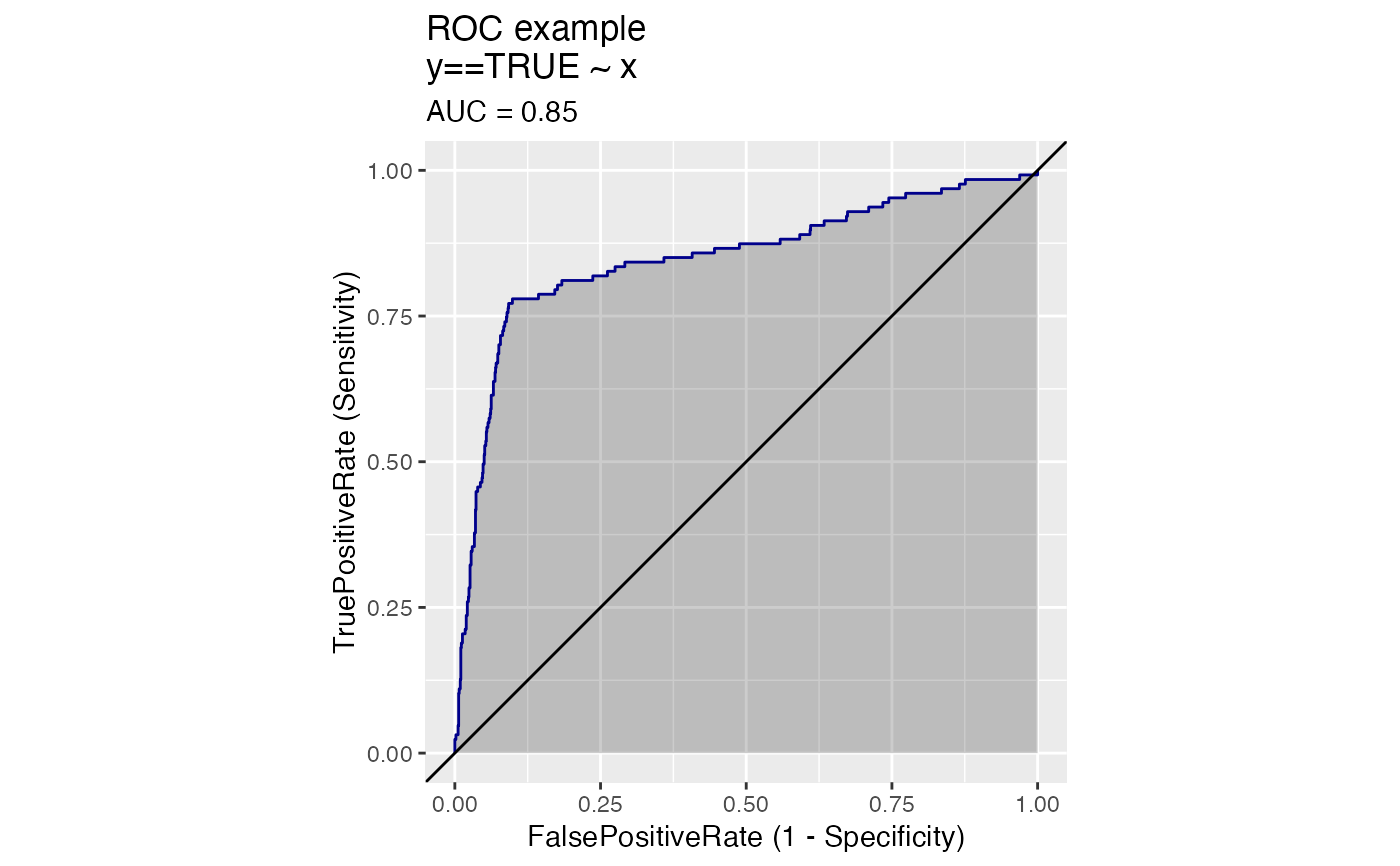# Precision/Recall examples
ThresholdPlot(d, 'x', 'y',
title = 'precision/recall',
metrics = c('recall', 'precision'),
truth_target = TRUE)
#> Warning: Removed 1 row(s) containing missing values (geom_path).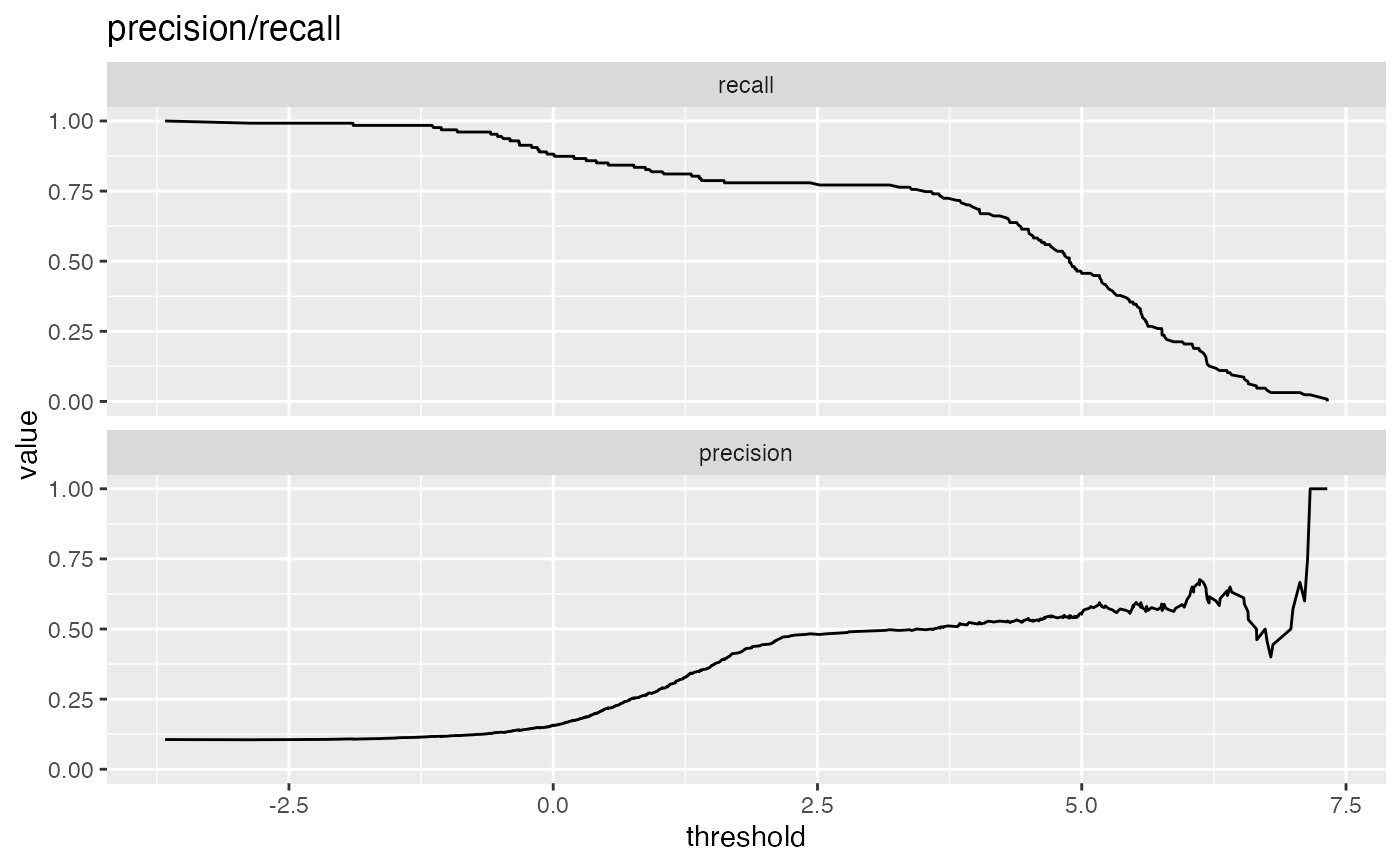MetricPairPlot(d, 'x', 'y',
x_metric = 'recall',
y_metric = 'precision',
title = 'recall/precision',
truth_target = TRUE)
#> Warning: Removed 2 row(s) containing missing values (geom_path).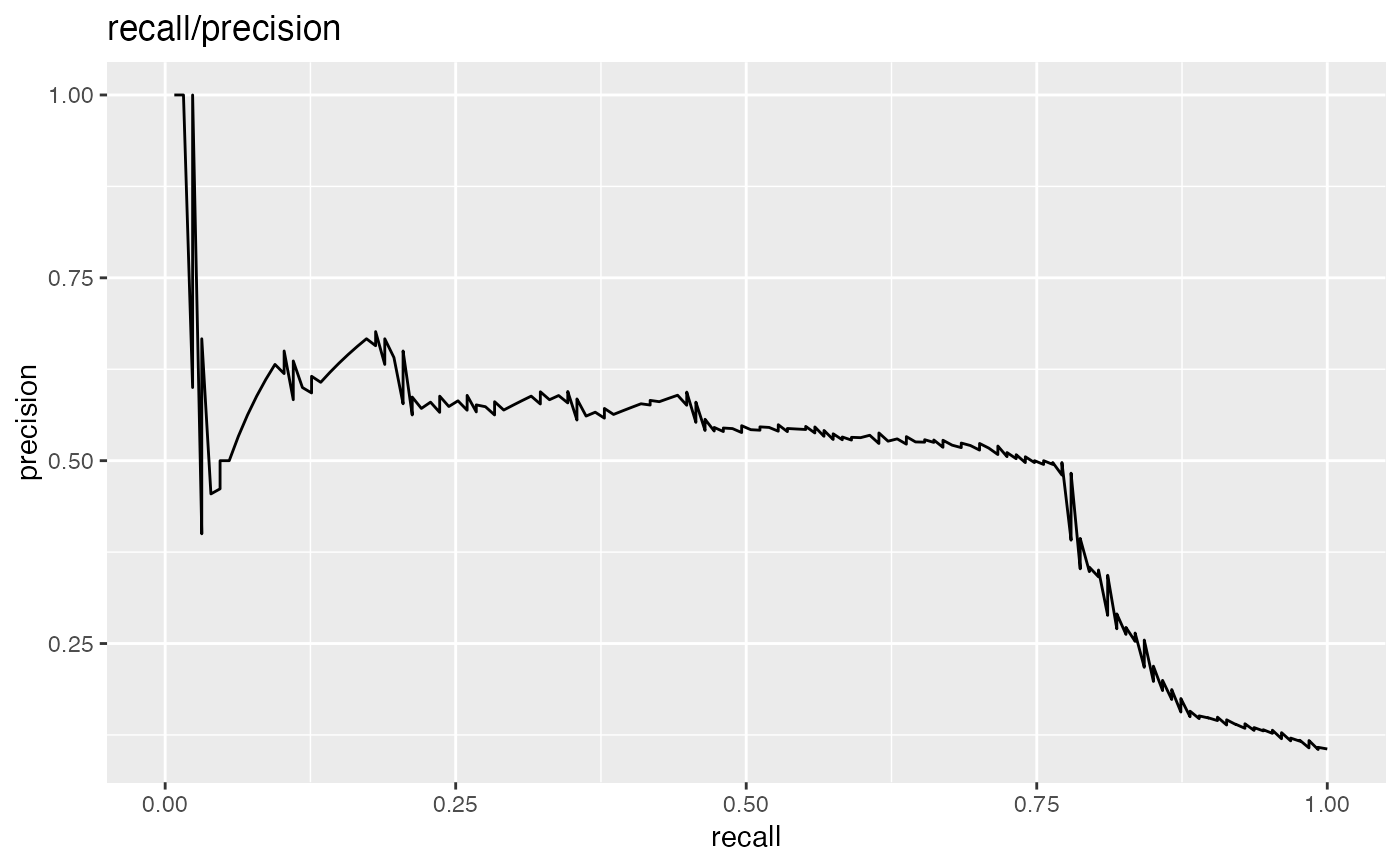PRPlot(d, 'x', 'y',
truthTarget = TRUE,
title = 'p/r plot')